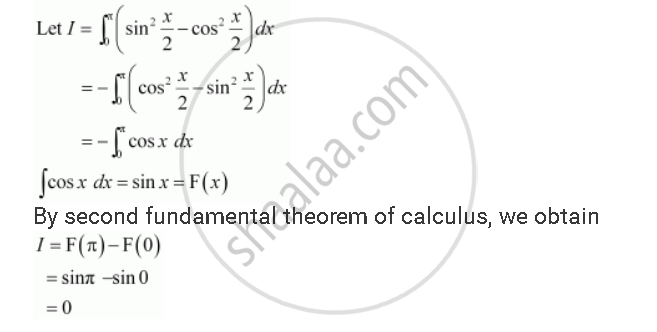Share

# Evaluate the Definite Integrals Int_0^Pi (Sin^2 X/2 - Cos^2 X/2) Dx - CBSE (Commerce) Class 12 - Mathematics

#### Question

Evaluate the definite integrals int_0^Pi (sin^2 x/2 - cos^2 x/2) dx

#### SolutionIs there an error in this question or solution?

#### Video TutorialsVIEW ALL 

Solution Evaluate the Definite Integrals Int_0^Pi (Sin^2 X/2 - Cos^2 X/2) Dx Concept: Fundamental Theorem of Calculus.
S